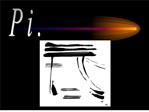# Exploring p - PowerPoint PPT PresentationDownload PresentationExploring p

Exploring p
Download Presentation## Exploring p

- - - - - - - - - - - - - - - - - - - - - - - - - - - E N D - - - - - - - - - - - - - - - - - - - - - - - - - - -
##### Presentation Transcript

3. Exploring p The measurement of the inside of a circle is its

4. The Radius The radius is a line segment that goes from the center point to any point on your circle. r = radius.

5. The diameter The diameter is a line segment that passes through the center point of your circle extending to two opposite points on the circle. d = diameter.

6. p Can you say p? Pi is a number with infinite decimal places we use to find the circumference and area of circles.

7. We have many formulas with p

8. Here is the estimate of pi that most people use.

9. Divide the circumference by the diameter

10. Divide the circumference by the diameter

11. Divide the circumference by the diameter

18. Let�s take a look at pi. p goes on forever and ever! How long would it take to enter that on a calculator? We use a shortened version of p.. Here�s part of pi���...

19. Are you ready? Do you want to use pi? 3.14159265358979323846264338327950288419716939937510582097494459230781640628620899862803482534211706798214808651328230664709384460955058223172535940812848111745028410270193852110555964462294895493038196442881097566593344612847564823378678316527120190914564856692346034861045432664821339360726024914�.

20. Again, pi goes on forever and ever... Mathematicians have calculated the first 6 billion digits in pi. Your school calculator has a p key that is the first 10 digits. 3.141592654 We usually use the first three digits if we do not have a calculator handy.

22. There is no end to pi. But, this is the end of this show.